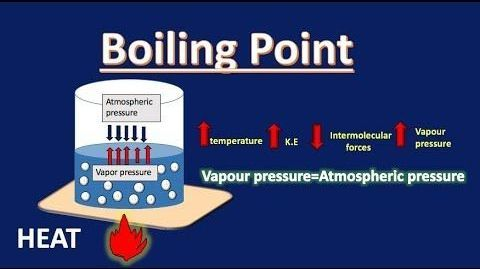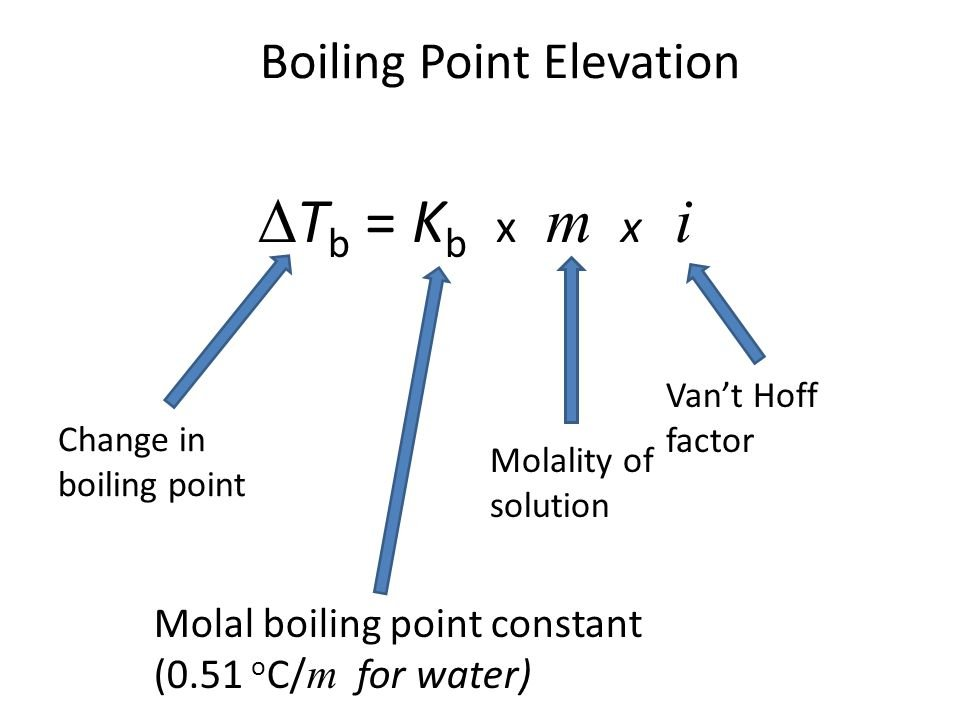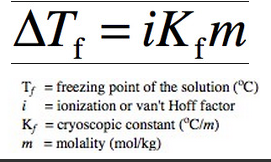# Boiling Point Elevation and Freezing Point Depression Study Guide

INTRODUCTION

We have learned that water boils at 100°C all of the time; however, this is not entirely true. The water temperature when it boils varies based on where you are and what you add to it. The addition of salt to water is one such example. In our cooking, salt is a fantastic element. It enhances the flavor of our dish and increases the boiling point of water. This is an example of the elevation of the boiling point of a liquid. The boiling point rises with the amount of salt added because it is a colligative feature dependent on the number of particles generated in the liquid. We will discuss boiling point elevation and freezing point depression in this article.Source

### COLLIGATIVE PROPERTIES

Colligative properties in chemistry are qualities of liquids determined by the proportion of solute to solvent particles in a solution, rather than the character of the chemical species present.

### BOILING POINT ELEVATION

Boiling point elevation occurs when the boiling point of a solution exceeds the boiling point of a pure solvent. The addition of solute to a solvent causes the vapor pressure of the solution to drop below that of the pure solvent, causing the solution’s boiling point to rise above that of the pure solvent. It’s a colligative property, which means it’s determined by the number of particles in a solution rather than the kind or weight of those particles. The most typical application of this phenomenon is the above-discussed example, the addition of salt to the water to raise the boiling point. The formula for boiling point elevation is:Source

### FREEZING POINT DEPRESSION

If we add a solute to a solvent, a few molecules occupy space on the liquid’s surface. Solvent molecules have to be available at the solution’s surface to evaporate, as we all know. Because the existence of solute molecules at the surface reduces the surface area available to solvent molecules, the solvent vaporization rate is reduced. As a result, adding solute molecules lowers the vapor pressure of the solution, which decreases the freezing point of a solution, which is referred to as “freezing point depression.” It is the difference in temperature between the freezing points of the pure solvent and the solution. It’s a colligative quality seen in solutions formed by the addition of solute molecules. The formula for freezing point depression is:Source

## CONCLUSION

• Boiling point elevation and freezing point depression are colligative properties of a solution.
• The amount by which the boiling point rises on the addition of a solute may be computed using the boiling point elevation constant and the molality of the solution.
• Using the Freezing point depression constant and the molality of the solution, the amount by which the decrease in freezing point decreases may be computed.

### FAQs

1. How do you calculate boiling-point elevation?

The following is a simple equation for determining the elevation of a solution’s boiling point: mKb = ΔT ( elevation in boiling-point)

2. What is the formula for boiling point elevation and freezing point depression?

The formula for boiling point elevation is mKb = ΔTb